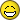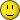6 Posts

## Subject: Confuse over how to read d100 and d10In Panzer game, we roll two d10 die. One with white and another one with color. We have to pre-determine which one will be the d100 and which will be d10. If I rolled 0 for d100 die and 8 for d10 die, it should read 08 or 108 for the result?

0 is read as 10 for d10, so 0 should be read as 100 for d100?
• [+] Dice rolls
The range of possible outcomes should be 1-100 (although some games might use 0 to 99).

When the range is 1 to 100, it's pretty simple to figure out what must be what. Let's assume a white die is the tens digit, and the black die is the ones digit, so that the expression W4B5 means you rolled a "45".

In that case, a W0B8 would be "8", as the highest value one can roll is "100". Accordingly, W0B0 would be "100". W1B0 would be "10", W9B0 would be "90", W9B9 would be "99", and so on.

If you're playing a game where the desired range is 0-99, instead, then 0 on both dice is zero, so that W0B0 is zero, W0B1 is one, and W9B9 is 99.
• [+] Dice rolls
Reference section 3.2 of the rules on how to read the dice. A roll (100) can only produce a result from 1 to 100. The final modified result can be less than 1 or greater than 100.
• [+] Dice rolls
Thank you very much guys! You safe my day!• [+] Dice rolls
Erathor wrote:
In Panzer game, we roll two d10 die. One with white and another one with color. We have to pre-determine which one will be the d100 and which will be d10. If I rolled 0 for d100 die and 8 for d10 die, it should read 08 or 108 for the result?

0 is read as 10 for d10, so 0 should be read as 100 for d100?
When rolling 1 die, the '0' is 10.

When rolling two dice, the '0' is 0: unless, however, you roll two '0''s, in which case the reading is 100 rather than 0.

So, with two dice, '4' and '0' is 40. '0' and '4' is 4. '0' and '0', however, is 100.

• [+] Dice rolls
Thank you Robert!• [+] Dice rolls